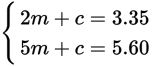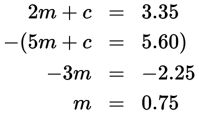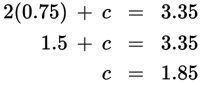# SAT Math Multiple Choice Question 467: Answer and Explanation

### Test Information

Question: 467

2. Two muffins and a carton of milk cost \$3.35. If five muffins and a carton of milk cost \$5.60, what is the cost of two cartons of milk?

• A. \$0.75
• B. \$1.50
• C. \$1.85
• D. \$3.70

Explanation:

D

Difficulty: Medium

Category: Heart of Algebra / Systems of Linear Equations

Strategic Advice: Write a system of equations with m = the number of muffins and c = the number of cartons of milk. Before you choose your answer, make sure you answered the right question (the cost of two cartons of milk).

Getting to the Answer: Translate from English into math to write the two equations: The first statement is translated as 2m + c = \$3.35 and the second as 5m + c = \$5.60. The system isYou could solve the system using substitution, but elimination is quicker in this question, because subtracting the second equation from the first eliminates c, and you can solve for m:Substitute this value for m in the first equation, and solve for c:So two cartons of milk would cost 2 × \$1.85 = \$3.70.